# HBSE 10th Class Maths Solutions Chapter 7 Coordinate Geometry Ex 7.1

Haryana State Board HBSE 10th Class Maths Solutions Chapter 7 Coordinate Geometry Ex 7.1 Textbook Exercise Questions and Answers.

## Haryana Board 10th Class Maths Solutions Chapter 7 Coordinate Geometry Ex 7.1

Question 1.
Find the distance between the following pairs of points :
(i) (2, 3), (4, 1)
(ii) (- 5, 7), (- 1, 3)
(iii) (a, 6), (- a, – b)
Solution :
(i) Let the given points be A(2, 3) and B(4, 1), then
AB = $$\sqrt{(4-2)^2+(1-3)^2}$$
⇒ AB = $$\sqrt{(2)^2+(-2)^2}$$
⇒ AB = $$\sqrt{4+4}$$
⇒ AB = √8
⇒ AB = $$\sqrt{2 \times 2 \times 2}$$
⇒ AB = 2√2
Hence, distance (AB) = 2√2.

(ii) Let the given points be P(- 5, 7) and Q(- 1, 3), then
PQ = $$\sqrt{(-1+5)^2+(3-7)^2}$$
PQ = $$\sqrt{(4)^2+(-4)^2}$$
⇒ PQ = $$\sqrt{16+16}$$
⇒ PQ = √32
⇒ PQ = $$\sqrt{4 \times 4 \times 2}$$
⇒ PQ = 4√2
Hence, distance (PQ) = 4√2

(iii) Let the given points be P(a, b) and Q(- a, – 6), then
PQ = $$\sqrt{(-a-a)^2+(-b-b)^2}$$
⇒ PQ = $$\sqrt{(-2 a)^2+(-2 b)^2}$$
⇒ PQ = $$\sqrt{4 a^2+4 b^2}$$
⇒ PQ = $$\sqrt{4\left(a^2+b^2\right)}$$
⇒ PQ = 2$$\sqrt{a^2+b^2}$$
Hence, the distance (PQ) = 2$$\sqrt{a^2+b^2}$$.Question 2.
Find the distance between the points (0,0) and (36,15). Can you now find the distance between the two towns A and B.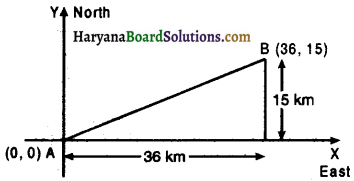Solution:
Let the given points be A(0,0) and B(36, 15) then
AB = $$\sqrt{(36-0)^2+(15-0)^2}$$
⇒ AB = $$\sqrt{(36)^2+(15)^2}$$
⇒ AB = $$\sqrt{1296+225}$$
⇒ AB = $$\sqrt{1521}$$
⇒ AB = 39
Hence, distance between two towns = 39 km.

Question 3.
Determine if the points (1, 5), (2, 3) and (- 2, – 11) are collinear.
Solution:
Let the given points be A(1, 5), B(2, 3) and C(- 2, – 11). Then,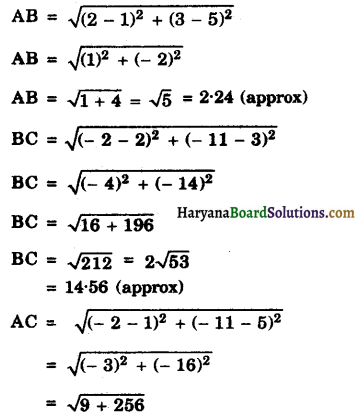⇒ AC = √256 = 16.28 (approx)
Now,AB + BC = 2.24 + 14.56 = 16.8
AC = 16.28
∴ AB + BC ≠ AC
Hence, the given points (1, 5), (2, 3) and (- 2, – 11) are not collinear.Question 4.
Check whether (5, – 2), (6,4) and (7, – 2) are the vertices of an isosceles triangle.
Solution :
Let the given points be A(5, – 2), B(6, 4) and C(7, – 2), then
AB = $$\sqrt{(6-5)^2+(4+2)^2}$$
= $$\sqrt{(1)^2+(6)^2}$$
= $$\sqrt{37}$$

BC = $$\sqrt{(7-6)^2+(-2-4)^2}$$
= $$\sqrt{(1)^2+(-6)^2}$$
= $$\sqrt{37}$$
Since, AB = BC
Therefore, the given joints are the vertices of an isosceles triangle.

Question 5.
In a class room, 4 friends are seated at the points A, B, C and D as shown in figure. Champa and Chameli walk into the class and after observing for a few minutes Champa asks Chameli, “Don’t you think ABCD is a square ?” Chameli disagrees. Using distance formula, find which of them is correct ?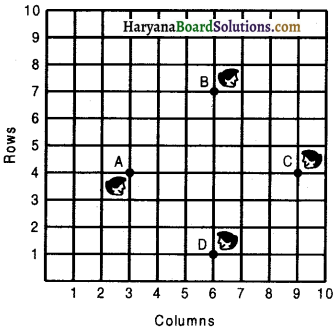Solution:
From the figure, coordinates of A, B, C, D, are respectively (3, 4), (6, 7), (9, 4), (6, 1)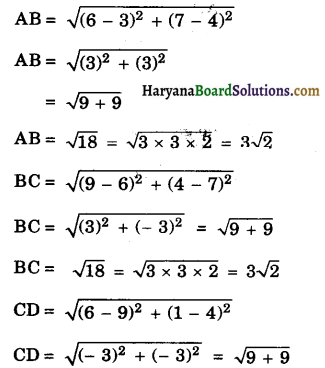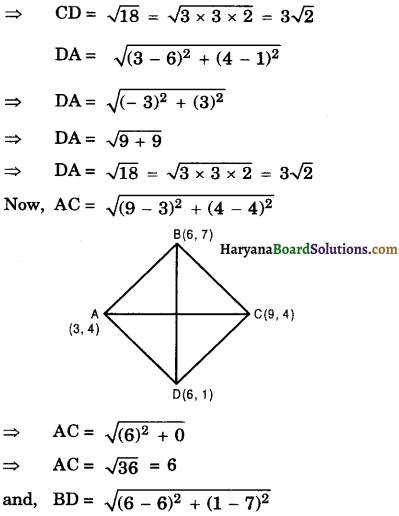⇒ BD = √36
⇒ BD = 6.
Since, AB = BC = CD = DA and diagonal AC = diagonal BD.
Therefore, ABCD is a square.
Hence, Champa is correct.Question 6.
Name the type of quadrilateral formed, if any, by the following points, and give reasons for your answer :
(i) (- 1, – 2), (1, 0), (- 1, 2), (- 3, 0)
(ii) (- 3, 5), (3, 1), (0, 3), (-1, – 4)
(iii) (4, 6), (7, 6), (4, 3), (1, 2)
Solution :
(i) Let the given points of the quadrilateral be A(- 1, – 2), B(1, 0), C(- 1, 2) and D(- 3, 0).
Join AC and BD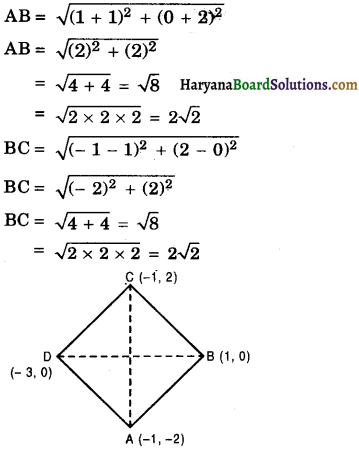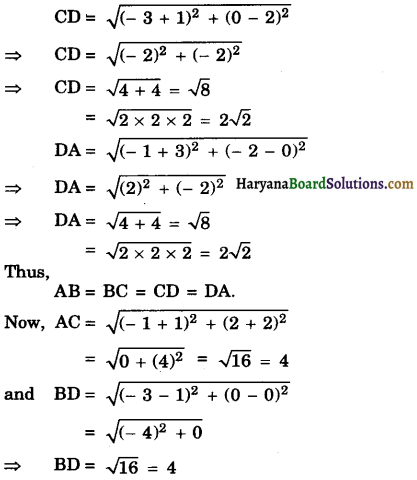Since, AB = BC = CA = DA and diagonal AC = diagonal BD
Therefore, given points form a square.(ii) Let the given points of the quadrilateral be A(- 3, 5), B(3, 1), C(0, 3) and D(- 1, – 4).
Join AC and BD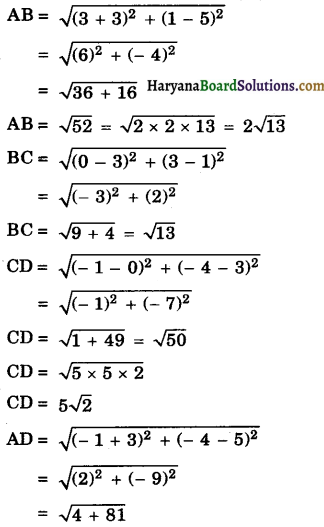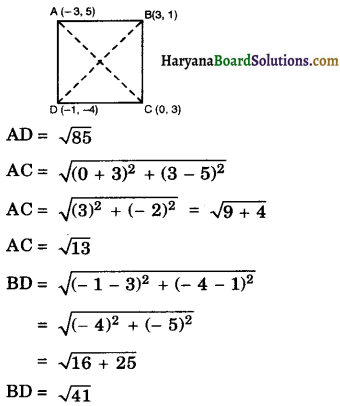Now,
AC + BC = √13 + √13 = 2√13
AB = 2√13
Since, AC + BC = AB.
Therefore, A, B, C are collinear. So, the given points do not form any quadrilateral.(iii) The given points of a quadrilateral be A(4, 5), B(7, 6), C(4, 3) and D(l, 2). Join AC and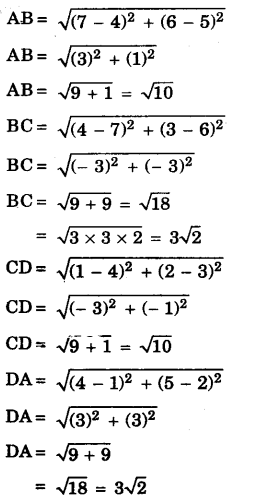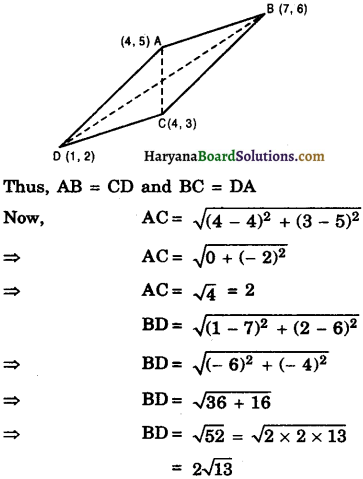Diagonal AC ≠ diagonal BD.
Since, opposite sides are equal but diagonals are not equal.
Therefore, the given points form a parallelogram.Question 7.
Find the point on the x-axis which is equidistant from (2, – 5) and (- 2, 9).
Solution :
Let the given points be A(2, – 5) and B(- 2, 9) and let the required point be POc, 0), then
PA = PB
⇒ PA2 = PB2
⇒ (x – 2)2 + (0 + 5)2 = (x + 2)2 + (0 – 9)2
⇒ x2 + 4 – 4x + 25 = x2 + 4 + 4x + 81
⇒ x2 – 4x + 29 = x2 + 4x + 85
⇒ x2 – 4x – x2 – 4x = 85 – 29
⇒ – 8x = 56.
⇒ x = $$\frac{56}{-8}$$
x = – 7
Hence, the required point = (- 7, 0)

Question 8.
Find the values ofy for which the distance between the points P(2, – 3) and Q(10, y) is 10 units.
Solution :
The given points are P(2, – 3) and Q(dfgvbtyh10, y)
and PQ = 10 units
∴ PQ = $$\sqrt{(10-2)^2+(y+3)^2}$$
10 = $$\sqrt{(8)^2+y^2+9+6 y}$$
10 = $$\sqrt{64+y^2+9+6 y}$$
10 = $$\sqrt{73+y^2+6 y}$$
100 = 73 + y2 + 6y (squaring both sides)
y2 + 6y + 73 – 100 = 0
y2 + 6y – 27 = 0
y2 + (9 – 3)y – 27 = 0
y2 + 9y – 3y – 27 = 0
[∵ 1 × – 27 = – 27
9 × – 3 = – 27
9 – 3 = 6]
⇒ y(y + 9) – 3(y + 9) = 0
⇒ (y + 9) (y – 3) = 0
⇒ y + 9 = 0 or y – 3 = 0
⇒ y = – 9 or y = 3.
Hence, y = – 9 or 3.Question 9.
If Q(0, 1) is equidistant from P(5, – 3) and R(x, 6), find the values of x. Also find the distances QR and PR.
Solution:
The given points are P(5, -3), Q(0, 1) and R(x, 6)
∵ Q is the equidistant from P and R.
∴ PQ = RQ
⇒ PQ2 = RQ2
⇒ (0 – 5)2 + (1 + 3)2 = (0 – x)2 + (1 – 6)2
⇒ (- 5)2 + (4)2 = (- x)2 + (- 5)2
⇒ 25 + 16 = x2 + 25
⇒ 25 – 25 + 16 = x2
⇒ x2 = 16
⇒ x = ± 4

Case II: x = 4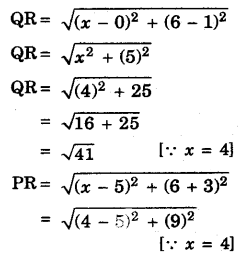PR = $$\sqrt{(-1)^2+81}$$
PR = $$\sqrt{1+81}=\sqrt{82}$$

Case II: If x = – 4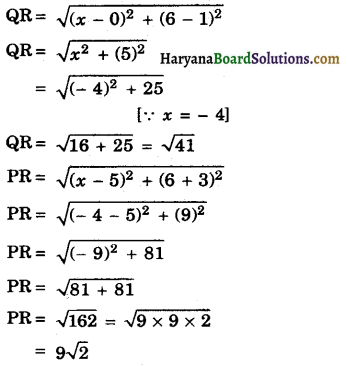Hence, x = ± 4, QR = √41 and PR = √82 or 9√2.

Question 10.
Find a relation between x and y such that the point (x, y) is equidistant from the points (3, 6) and (-. 3, 4).
Solution:
Let P(x, y) be the equidistant from the points M(3, 6) and B(- 3, 4), then
PA = PB
PA2 = PB2 .
⇒ (3 – x)2 + (6 – y)2
⇒ (- 3 – x)2 + (4 – y)2
⇒ 9 + x2 – 6x + 36 + y2 – 12y = 9 + x2 + 6x + 16 + y2 – 8y
⇒ x2 + y2 + 6x – 8y + 6x + 12y – x2 -y2 = 45 – 25
⇒ 12x + 4y = 20
⇒ 3x + y = 5
⇒ 3x + y – 5 = 0
Hence, the required relation is 3x + y – 5 = 0